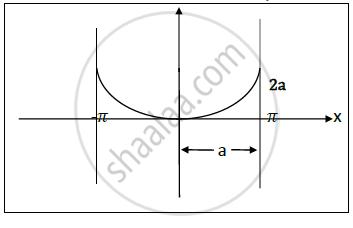# Find the Length of Cycloid from One Cusp to the Next , Where X = a ( θ + Sin θ ) , Y = a ( 1 − Cos θ ) - Applied Mathematics 2

Find the length of cycloid from one cusp to the next , where x=a(θ + sinθ) , y=a(1-cosθ)

#### Solution

Given curve :Cycloid x=a(θ + sinθ) , y=a(1-cosθ)The length of given curve is :

s=int_(θ1)^(θ2) sqrt((dx/(dθ))+(dy/(dθ))^2 dθ)

dx/(dθ)=a(1+cosθ)                dy/(dθ)=a sin θ

∴ (dx/(dθ))^2 +(dy/(dθ))^2=a^2[1+2 cosθ +cos^2 θ+sin^2θ ]

=2a^2[1+cosθ]

= 4a^2 [cos^2  θ/2]

∴sqrt((dx/dθ)^2+(dy/(dθ))^2)= 2a cos  θ/2

∴ s= int_-pi^pi 2acos  θ/2  dθ

= 2xxint_0^pi 2 a cos  θ/2 dθ

= 4a [2 sin  θ/2]_0^pi

∴ s= 8a

Concept: Linear Differential Equation with Constant Coefficient‐ Complementary Function
Is there an error in this question or solution?